Linear Combination of Vectors Next  |  Prev  |  Up  |  Top  |  Index  |  JOS Index  |  JOS Pubs  |  JOS Home  |  Search

## Linear Combination of Vectors

A linear combination of vectors is a sum of scalar multiples of those vectors. That is, given a set of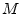vectors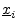of the same type,5.4 such as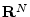(they must have the same number of elements so they can be added), a linear combination is formed by multiplying each vector by a scalar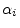and summing to produce a new vector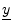of the same type: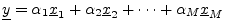For example, let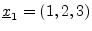,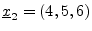,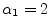, and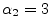. Then the linear combination of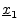and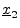is given by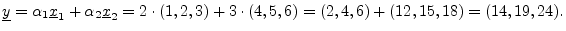In signal processing, we think of a linear combination as a signal mix. Thus, the output of a mixing console may be regarded as a linear combination of the input signal tracks.

Next  |  Prev  |  Up  |  Top  |  Index  |  JOS Index  |  JOS Pubs  |  JOS Home  |  Search

[How to cite this work] [Order a printed hardcopy]